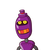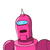# the cost price and selling price of an article is in the ratio a:b. If b is 200% of a, then the percentage of profit on cost price

the cost price and selling price of an article is in the ratio a:b. If b is 200% of a, then the percentage of profit on cost price is​

### 2 thoughts on “the cost price and selling price of an article is in the ratio a:b. If b is 200% of a, then the percentage of profit on cost price”

1.100%

Step-by-step explanation:

a= c.p and b= s.p

if s.p or b is 200% of a or c.p, then

s.p= 200% of c.p

= (200× a or c.p)/100

=2a

so s.p= double of cost price

now as s.p = 2a

and ç.p= a

the profit= s.p-c.p

profit= 2a- a= a

now to find profit %

profit % = (profit/c.p)× 100%

profit %= ( a/a)× 100%

profit %=100%

2.